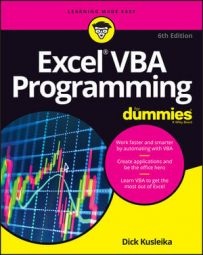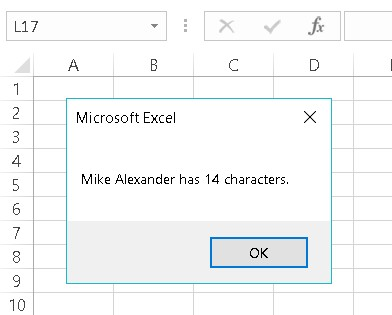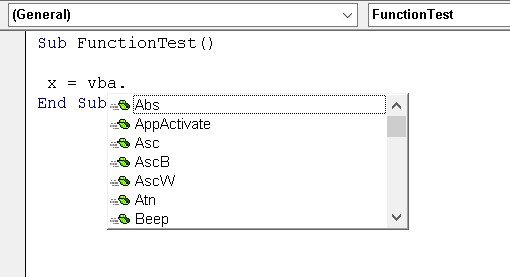##### Excel VBA Programming For DummiesExcel VBA provides numerous built-in functions. Some of these Excel VBA functions take arguments, and some do not.

## Excel VBA function examples

Here, you find a few examples of using VBA functions in code. In many of these examples, the MsgBox function displays a value in a message box. Yes, MsgBox is a VBA function — a rather unusual one, but a function nonetheless. This useful function displays a message in a dialog box and also returns a value.

### Displaying the system date or time

The first example uses VBA’s Date function to display the current system date in a message box:
```Sub ShowDate()
MsgBox "Today is: " & Date
End Sub```
Notice that the Date function doesn’t use an argument. Unlike worksheet functions, a VBA function with no argument doesn’t require an empty set of parentheses. In fact, if you type an empty set of parentheses, the VBE promptly removes them.

To get the system time, use the Time function. And if you want it all, use the Now function to return both the date and the time.

### Finding a string length

The following procedure uses the VBA Len function, which returns the length of a text string. The Len function takes one argument: the string. When you execute this procedure, the message box displays your name, and the number of characters in your name.
```Sub GetLength()
Dim MyName As String
Dim StringLength As Long
StringLength = Len(MyName)
MsgBox MyName & " has " & StringLength & " characters."
End Sub```Calculating the length of your name.

Excel also has a LEN function, which you can use in your worksheet formulas. The Excel version and the VBA function work the same.

### Displaying the name of a month

The following procedure uses the MonthName function, which returns the name of a month. MonthName uses one argument: an integer between 1 and 12.
```Sub ShowMonthName()
Dim ThisMonth As Long
ThisMonth = Month(Date)
MsgBox MonthName(ThisMonth)
End Sub```
This procedure uses the Month function to get the current month (as a value), and this value is assigned to the ThisMonth variable. The MonthName function then converts the value to text. So if you run this procedure in April, the message box displays the text April.

Actually, the ThisMonth variable isn’t required. You can get the same effect with this expression, which uses three VBA functions:

`MonthName(Month(Date))`
Here, the current date is passed as an argument to the Month function, which returns a value that’s passed as an argument to the MonthName function.

### Determining a file size

The following Sub procedure displays the size, in bytes, of the Excel executable file. It finds this value by using the FileLen function:
```Sub GetFileSize()
Dim TheFile As String
TheFile = "C:\Program Files (x86)\Microsoft Office\root\Office16\EXCEL.EXE"
MsgBox FileLen(TheFile)
End Sub```
Notice that this routine hard-codes the filename (that is, explicitly states the path). This isn’t a good idea. The file may not be on the C drive, or the Excel folder may have a different name. The following statement shows a better approach:
`TheFile = Application.Path & "\EXCEL.EXE"`
Path is a property of the Application object. It simply returns the name of the folder in which the application (that is, Excel) is installed (without a trailing backslash).

### Identifying the type of a selected object

The following procedure uses the TypeName function, which returns the type of the selection on the worksheet (as a string):
```Sub ShowSelectionType()
Dim SelType As String
SelType = TypeName(Selection)
MsgBox SelType
End Sub```
The selection could be a Range, a Picture, a Rectangle, a ChartArea, or any other type of object that can be selected.

The TypeName function is very versatile. You can also use this function to determine the data type of a variable.

## Excel VBA functions that do more than return a value

A few VBA functions go above and beyond the call of duty. Rather than simply return a value, these functions have some useful side effects.
 Function What It Does MsgBox Displays a handy dialog box containing a message and buttons. The function returns a code that identifies which button the user clicks. InputBox Displays a simple dialog box that asks the user for some input. The function returns whatever the user enters in the dialog box. Shell Executes another program. The function returns the task ID (a unique identifier) of the other program (or an error if the function can’t start the other program).

## Discovering Excel VBA functions

How do you find out which functions VBA provides? Good question. The best source is the Excel VBA system. Another way is to type VBA, followed by a period. You get a list of items. Those with a green icon are functions. If this feature isn’t working, choose VBE’s Tools → Options, click the Editor tab, and place a check next to Auto List Members.A way to display a list of VBA functions.

There are over 140 different functions available in VBA. Some are so specialized and obscure, you’ll never need them. Others, however, are quite useful for many applications.

 Function What It Does Abs Returns a number’s absolute value Array Returns a variant containing an array Choose Returns a value from a list of items Chr Converts an ANSI value to a string CurDir Returns the current path Date Returns the current system date DateAdd Returns a date to which a specified time interval has been added — for example, one month from a particular date DateDiff Returns an integer showing the number of specified time intervals between two dates — for example, the number of months between now and your birthday DatePart Returns an integer containing the specified part of a given date — for example, a date’s day of the year DateSerial Converts a date to a serial number DateValue Converts a string to a date Day Returns the day of the month from a date value Dir Returns the name of a file or directory that matches a pattern Err Returns the error number of an error condition Error Returns the error message that corresponds to an error number Exp Returns the base of the natural logarithm (e) raised to a power FileLen Returns the number of bytes in a file Fix Returns a number’s integer portion Format Displays an expression in a particular format GetSetting Returns a value from the Windows registry Hour Returns the hour portion of a time InputBox Displays a box to prompt a user for input InStr Returns the position of a string within another string (counting from the start) InStrRev Returns the position of a string within another string (counting from the end) Int Returns the integer portion of a number IsArray Returns True if a variable is an array IsDate Returns True if an expression is a date IsEmpty Returns True if a variable has not been initialized IsError Returns True if an expression is an error value IsMissing Returns True if an optional argument was not passed to a procedure IsNull Returns True if an expression contains no valid data IsNumeric Returns True if an expression can be evaluated as a number LBound Returns the smallest subscript for a dimension of an array LCase Returns a string converted to lowercase Left Returns a specified number of characters from the left of a string Len Returns the number of characters in a string Mid Returns a specified number of characters from a string Minute Returns the minutes portion of a time value Month Returns the month from a date value MsgBox Displays a message box and (optionally) returns a value Now Returns the current system date and time Replace Replaces a substring in a string with another substring RGB Returns a numeric RGB value representing a color Right Returns a specified number of characters from the right of a string Rnd Returns a random number between 0 and 1 Second Returns the seconds portion of a time value Shell Runs an executable program Space Returns a string with a specified number of spaces Split Splits a string into parts, using a delimiting character Sqr Returns a number’s square root String Returns a repeating character or string Time Returns the current system time Timer Returns the number of seconds since midnight TimeSerial Returns the time for a specified hour, minute, and second TimeValue Converts a string to a time serial number Trim Returns a string without leading or trailing spaces TypeName Returns a string that describes a variable’s data type UBound Returns the largest available subscript for an array’s dimension UCase Converts a string to uppercase Val Returns the numbers contained in a string Weekday Returns a number representing a day of the week Year Returns the year from a date value

For complete details on a particular Excel VBA function, type the function name in a VBA module, move the cursor anywhere in the text, and press F1.